# Phenol Red - pH Indicator

#### 2022-05-03

Phenol red is an indicator commonly used to measure pH in swimming pool test kits, see e.g. . The goal of this colorSpec vignette is to reproduce the colors seen in such a test kit, for typical values of pool pH. Calculations like this one might make a good project for a college freshman chemistry class. Featured functions in this vignette are: interpolate() and calibrate().

library( colorSpec )
library( spacesRGB )    # for functions plotPatchesRGB() and SignalRGBfromLinearRGB()

## Absorbance Spectra at Different pH Values

The absorbance data for phenol red has already been digitized from :

path = system.file( "extdata/stains/PhenolRed-Fig7.txt", package="colorSpec" )
wave = 350:650
phenolred = readSpectra( path, wavelength=wave )
par( omi=c(0,0,0,0), mai=c(0.6,0.7,0.4,0.2) )
plot( phenolred, main='Absorbance Spectra of Phenol Red at Different pH Values' )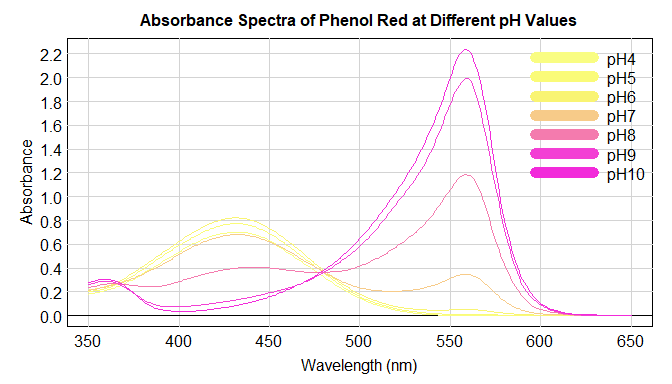Compare this plot with , Fig. 7. Unfortunately, the concentration and optical path length are unknown, but these curves can still be used as ‘relative absorbance’.

## Absorbance at Selected Wavelengths

We investigate how absorbance depends on pH for a few selected wavelengths.

wavesel = c( 365, 430, 477, 520, 560, 590 )  # 365 and 477 are 'isosbestic points'
mat = apply( as.matrix(wavesel), 1, function( lambda ) { as.numeric(lambda == wave) } )
colnames( mat ) = sprintf( "%g nm", wavesel )
mono = colorSpec( mat, wavelength=wave, quantity='power' )
RGB = product( mono, BT.709.RGB, wavelength=wave )  # this is *linear* RGB
colvec = grDevices::rgb( SignalRGBfromLinearRGB( RGB/max(RGB), which='scene' )$RGB ) phenolsel = resample( phenolred, wavesel ) pH = as.numeric( sub( '[^0-9]*([0-9]+)$', '\\1', specnames(phenolred) ) )
pHvec = seq(min(pH),max(pH),by=0.05)
phenolsel = interpolate( phenolsel, pH, pHvec )
mat = t( as.matrix( phenolsel ) )
par( omi=c(0,0,0,0), mai=c(0.8,0.9,0.6,0.4) )
plot( range(pH), range(mat), las=1, xlab='pH', ylab='absorbance', type='n' )
grid( lty=1 ) ; abline( h=0 )
matlines( pHvec, mat, lwd=3, col=colvec, lty=1 )
title( "Absorbance of Phenol Red at Selected Wavelengths")
legend( 'topleft', specnames(mono), col=colvec, lty=1, lwd=3, bty='n' )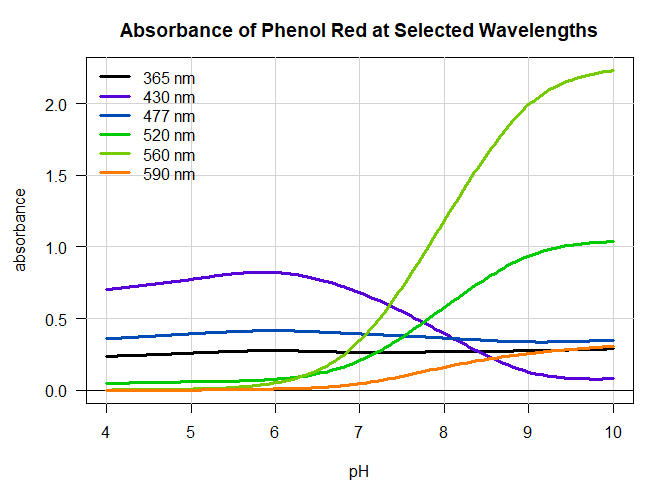Note that the curves for the isosbestic points 365 and 477 nm are approximately flat, as expected. But for 430 nm the curve is distinctly non-monotone. This indicates that the solution is not truly a mixture of the acidic and basic species (especially for pH $$\le$$ 6), and there may be an undesired side reaction, see .

## Interpolation from pH=6.8 to pH=8.2

Swimming pools should be slightly basic; a standard test kit covers the range from pH=6.8 to pH=8.2.

pHvec = seq(6.8,8.2,by=0.2)
phenolpool = interpolate( phenolred, pH, pHvec )
par( omi=c(0,0,0,0), mai=c(0.6,0.7,0.4,0.2) )
plot( phenolpool, main="Absorbance Spectra of Phenol Red at Swimming Pool pH Values" )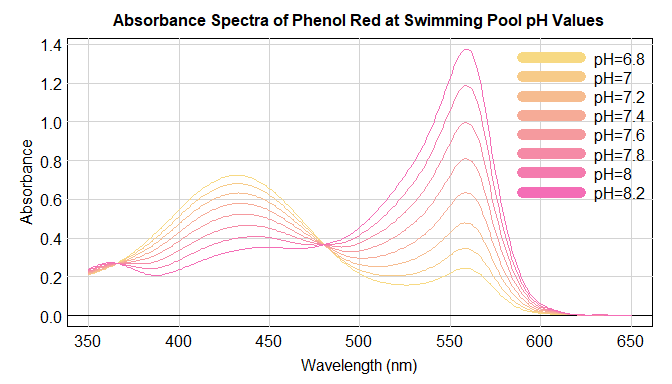The rest of this section is best viewed on a display calibrated for sRGB, see .

# create an uncalibrated 'material responder'
testkit = product( D65.1nm, 'solution', BT.709.RGB, wave=wave )
# now calibrate so that fully transparent pure water has response RGB=c(1,1,1)
testkit = calibrate( testkit, response=1 )
RGB = product( phenolpool, testkit )
RGB
##                R         G         B
## pH=6.8 1.0282473 0.6840105 0.2260205
## pH=7   1.0237233 0.5938036 0.2506686
## pH=7.2 1.0182971 0.4961330 0.2788955
## pH=7.4 1.0124869 0.4022827 0.3100400
## pH=7.6 1.0067433 0.3195781 0.3440245
## pH=7.8 1.0014227 0.2505541 0.3812950
## pH=8   0.9969035 0.1947707 0.4225796
## pH=8.2 0.9935212 0.1503867 0.4683717

Unfortunately, in some cases the red value is greater than 1 (G and B are OK). The color is outside the sRGB gamut. Start over and recalibrate.

testkit = product( D65.1nm, 'solution', BT.709.RGB, wave=wave )
# recalibrate, but lower the background a little, to allow more 'headroom' for indicator colors
bglin = 0.96  #  graylevel for the background, linear
testkit = calibrate( testkit, response=bglin )
RGB = product( phenolpool, testkit )   # this is *linear* sRGB
RGB
##                R         G         B
## pH=6.8 0.9871174 0.6566501 0.2169797
## pH=7   0.9827743 0.5700514 0.2406419
## pH=7.2 0.9775652 0.4762877 0.2677396
## pH=7.4 0.9719874 0.3861913 0.2976384
## pH=7.6 0.9664736 0.3067950 0.3302635
## pH=7.8 0.9613658 0.2405320 0.3660432
## pH=8   0.9570273 0.1869799 0.4056764
## pH=8.2 0.9537803 0.1443713 0.4496368

All values have been multiplied by bglin, and are now OK. Draw the RGB patches on a white background multiplied by the same amount.

df.RGB = data.frame( LEFT=1:nrow(RGB), TOP=0, WIDTH=1, HEIGHT=2 )
df.RGB$RGB = RGB par( omi=c(0,0,0,0), mai=c(0.3,0,0.3,0) ) plotPatchesRGB( df.RGB, space='sRGB', which='scene', labels=F, background=bglin ) text( (1:nrow(RGB)) + 0.5, 2, sprintf("%.1f",pHvec), adj=c(0.5,1.2), xpd=NA ) title( main='Calculated Colors for pH from 6.8 to 8.2' )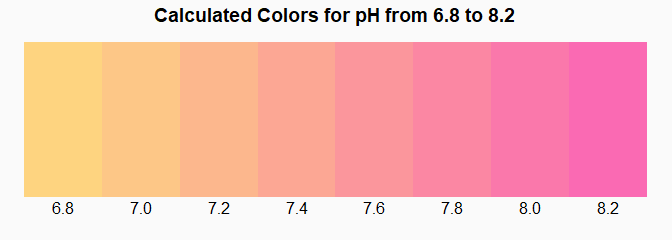The background color is that of pure water, and is not the full RGB=(255,255,255). In the first figure above, the phenol red concentration and optical path length are unknown. Compared to a real test kit, the calculated colors look a little faded. An absorbance multiplier can easily tweak the unknown concentration, as follows. tweak = 1.3 phenolpool = multiply( phenolpool, tweak ) df.RGB = data.frame( LEFT=1:nrow(RGB), TOP=0, WIDTH=1, HEIGHT=2 ) df.RGB$RGB = product( phenolpool, testkit ) # this is *linear scene* sRGB
par( omi=c(0,0,0,0), mai=c(0.3,0,0.3,0) )
plotPatchesRGB( df.RGB, space='sRGB', which='scene', background=bglin, labels=F )
text( (1:nrow(RGB)) + 0.5, 2, sprintf("%.1f",pHvec), adj=c(0.5,1.2), xpd=NA )
main = sprintf( 'Calculated Colors for pH from 6.8 to 8.2 (absorbance multiplier=%g)', tweak )
title( main=main )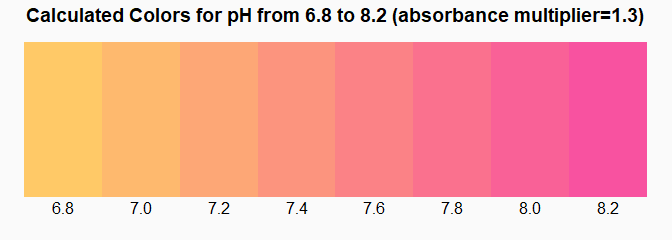These colors are a better match to those in the test kit.

## Session Information

R version 4.2.0 (2022-04-22 ucrt)
Platform: x86_64-w64-mingw32/x64 (64-bit)
Running under: Windows 10 x64 (build 19044)

Matrix products: default

locale:
 LC_COLLATE=C
 LC_CTYPE=English_United States.utf8
 LC_MONETARY=English_United States.utf8
 LC_NUMERIC=C
 LC_TIME=English_United States.utf8

attached base packages:
 stats     graphics  grDevices utils     datasets  methods   base

other attached packages:
 spacesRGB_1.4-0 colorSpec_1.4-0

loaded via a namespace (and not attached):
 digest_0.6.29        R6_2.5.1             jsonlite_1.8.0
 magrittr_2.0.3       evaluate_0.15        highr_0.9
 rlang_1.0.2          stringi_1.7.6        jquerylib_0.1.4
 bslib_0.3.1          rmarkdown_2.14       tools_4.2.0
 stringr_1.4.0        xfun_0.30            yaml_2.3.5
 fastmap_1.1.0        compiler_4.2.0       microbenchmark_1.4.9
 htmltools_0.5.2      knitr_1.39           sass_0.4.1